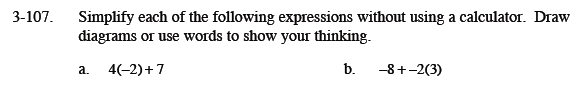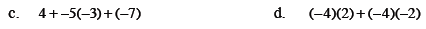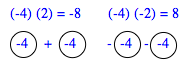### Home > MC1 > Chapter 3 > Lesson 3.4.2 > Problem3-107

3-107.
1. . Simplify each of the following expressions without using a calculator. Draw diagrams or use words to show your thinking. Homework Help ✎

1. 4(−2) + 7

2. −8 + −2(3)

3. 4 + −5(−3) + (−7)

4. (−4)(2) + (−4)(−2)Remember that terms are separated by addition symbols (+).

Can you draw or explain how you separate these terms?
You should simplify these terms before adding them together.

to draw a diagram like the tightropes used in previous lessons?

The product of this expression is −14.Reminder: Multiplying two negative numbers results in a positive number. Can you find the product and explain why this is so?

The expression simplifies to 12. Don't forget to show your thinking!

Mara used this picture to explain part of her reasoning. Does this help you to solve the problem?Can you think of another way to show your thinking?